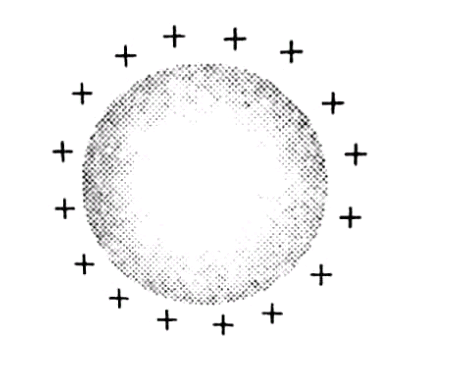Q

# Confused! kindly explain, There are two metallic spheres of same radii but one is solid and the other is hollow, then

There are two metallic spheres of same radii but one is solid and the other is hollow, then

• Option 1)

Solid sphere can be given more charge

• Option 2)

Hollow sphere can be given more charge

• Option 3)

They can be charged equally (maximum)

• Option 4)

None of the above

333 Views

As we learned

If surface is uniform -

Charge distributes uniformly.

- whereinBecause in case of metallic sphere either solid or hollow, the charge will reside on the surface of the sphere. Since both spheres have same surface area, so they can hold equal maximum charge.

Option 1)

Solid sphere can be given more charge

Option 2)

Hollow sphere can be given more charge

Option 3)

They can be charged equally (maximum)

Option 4)

None of the above

Exams
Articles
Questions